Eigen Values and Eigen Vectors

Diagonalisation

We mentioned earlier that it's much easier to multiply diagonal matrices. That means it's much easier to find what happens when a transformation with a diagonal matrix is repeated.
What is the fourth power of
 / 2 0 \ \ 0 1 /

We can quickly see the effect this transformation on a graph: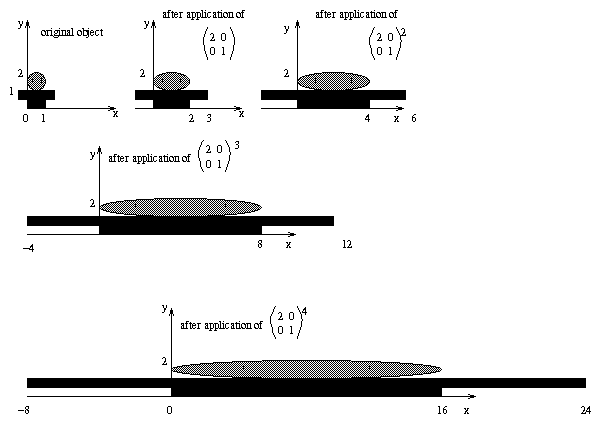how about finding the fourth power of
 / 3/2 1/2 \ \ 1/2 3/2 /

 / 2 -1 \ \ 0 1 /

Below is the effect of
 / 3/2 1/2 \ \ 1/2 3/2 /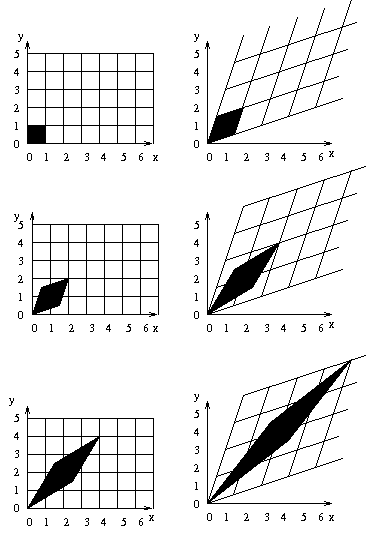I've drawn the effect of sucessive applications of the transformaion on the right. I've used the way the standard grid is transformed to work out where the succesive images must be. This is done by looking at the coordinates relative to the original grid; the transformed coordinates must have the same relation to the transformed grid. I'll explain this in class.
For the above graph, we can ask the following questions:
• What happens to the length of the diagonal of the "diamond" shape?
• write down the lengths of both diagonals for each of the pictures shown.
• Can you predict what the lengths of the diagonals will be after 5 steps?
• What will the coordiates of the points of the diamond after 5 steps?
• If we rotate by 45 degrees clockwise, how does it compare to the first transformation we looked at?
• Or what if we used a different grid to start with, at 45 degrees to the standard grid?

There are two directions which define a grid, which is transformed in a simple way: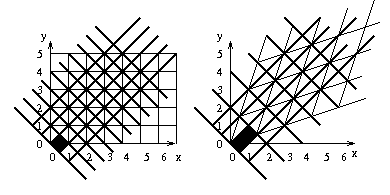How could we have found this grid without drawing the pictures?
If we could do that for other matices, we'd be able to see the effect of repeated transformations quickly. This is what diagonalisation is about.
The directions which define the grid are the eigen vectors, and the amounts of stretch in these directions are called the eigen values. So for
 / 3/2 1/2 \ \ 1/2 3/2 /
the eigen vectors are (1,1) and (1,-1), and the eigen values are 2,1.
For
 / 2 0 \ \ 0 1 /
the eigen vectors are (1,0) and (0,1), and the eigen values are 2,1.
There are a lot of problems were we want to find powers of matrices, so the eigen vectors and eigen values are very useful.
We will learn about how to find the eigen values and eigen vectors.
There will be lots of applications of these ideas.

Eigen Vectors

section 6.1

An eigen vector of a matrix A is a vector v such that A sends v to a multiple of itself. The multiple is called the eigen value corresponding to that vector. If the eigen value is L, we have
Av=Lv

If we know what an eigen value is, it's pretty easy to find what the corresponding eigen vector is. We did this in class, and it's covered in section 6.1 of the notes. All you have to do is find a non trivial solution of the equation
(A-LI)x=0
Here I is the identity matrix, and we are told what to use for L. This will be solved by row reduction of an augmented matrix, as usual.

Eigen Values

section 6.2

If A is an upper triangular matrix, then the eigen values must be the numbers occuring on the diagonal (see end of section 6.1).
How can we find the eigen values if the matrix is not upper triangular?
If A isn't upper triangular, we can start off in the same way, and we'll end up seeing that there is a polynomial called the characteristic polynomial, and it's roots are the eigen values. This polynomial is found using the determinant, which we'll also look at.
We will cover the population movement example again, from section 2.7, with a more sophisticated calculation now, in example 5 of section 6.2.

Diagonalization

section 6.3

Above, we saw what it means to find the diagonalization of a matrix, for an example. In general, to diagonalize a matrix A means that there is a matrix P, and a diagonal matrix D, with
A=PDP-1

Method of diagonalizing

• Find the eigen values, eg, a, b, c.
• Fird the corresponding eigen vectors, eg, u, v, w.
• Write down the matrix D, that's just got the eigen values on the diagaonal, the number of times an eigen value occurs is the same as the number of vectors you need to span that eigen space. eg:  / a 0 0 \ | 0 b 0 | \ 0 0 c /
• Write down the eigen vectors to get P, eg: (u,v,w)

Using diagonalizing to find powers of a matrix

Now we'll see how this helps us to find powers of matrices, and we'll see a method of finding the matrix P that diagonalizes A.
If
A=PDP-1
then
A8=PDP-1PDP-1PDP-1PDP-1PDP-1PDP-1PDP-1PDP-1

=PDDDDDDDDP-1

D8 is easy to find, so now we can find A8 easily too.

Diagonalization - Geometric interpretation

section 6.4

We will talk a little about what diagonalization means geometrically, with reference to the above example.

Calculating the eigenvalues/vectors

By definition,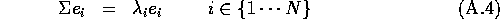Where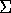represents the covariance matrix,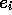represents the ith eigenvector, and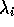is the ith eigenvalue.
for two-dimensions: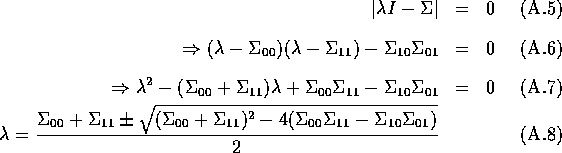This will find the two eigenvalues for the matrix. Similar reasoning can be used to find the eigenvalues of higher dimensional matrices.
Once we know the eigenvalues, the eigenvectorscan be calculated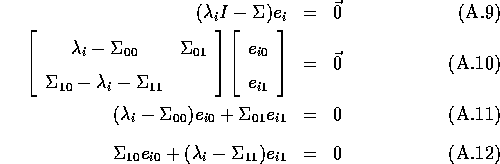Using Eqn A.11: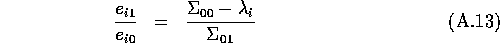This will lead to parametric vectors. Normalised vectors are typically used.